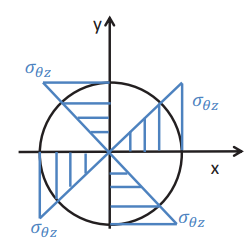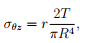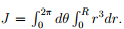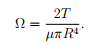# Circular Bar Civil Engineering (CE) Notes | EduRev

## Civil Engineering (CE) : Circular Bar Civil Engineering (CE) Notes | EduRev

The document Circular Bar Civil Engineering (CE) Notes | EduRev is a part of the Civil Engineering (CE) Course Advanced Solid Mechanics - Notes, Videos, MCQs & PPTs.
All you need of Civil Engineering (CE) at this link: Civil Engineering (CE)

Circular bar

As discussed, for illustration, we consider a bar with solid circular cross section of radius R, as shown in figure 9.6. From the general expression forFigure 9.7: Shear stresses in a bar with solid circular cross section subjected to end torsion

torsion of a thick walled close section (9.17), we find that the shear stresses in the bar is given by,(9.18)

where we have computedThus, we find that the shear stress varies linearly with the radial distance, as shown in figure 9.7.

Then, the angle of twist per unit length, Ω is also obtained from (9.17) as,(9.19)

Having determined the angle of twist per unit length, the entire displacement eld corresponding to an applied torque, T given by equation (9.8) is known.
The same is plotted in figure 9.8.

Offer running on EduRev: Apply code STAYHOME200 to get INR 200 off on our premium plan EduRev Infinity!

## Advanced Solid Mechanics - Notes, Videos, MCQs & PPTs

42 videos|61 docs

,

,

,

,

,

,

,

,

,

,

,

,

,

,

,

,

,

,

,

,

,

;